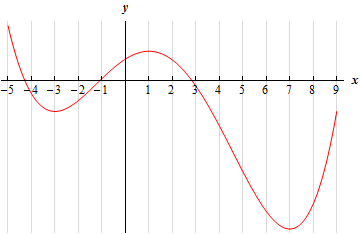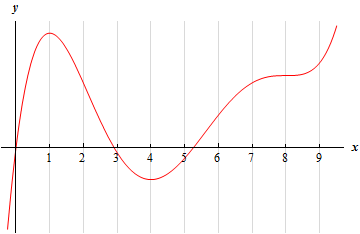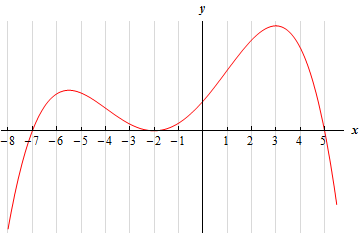Paul's Online Notes
Home / Calculus I / Applications of Derivatives / The Shape of a Graph, Part I
Show Mobile Notice Show All Notes Hide All Notes
Mobile Notice
You appear to be on a device with a "narrow" screen width (i.e. you are probably on a mobile phone). Due to the nature of the mathematics on this site it is best views in landscape mode. If your device is not in landscape mode many of the equations will run off the side of your device (should be able to scroll to see them) and some of the menu items will be cut off due to the narrow screen width.

### Section 4.5 : The Shape of a Graph, Part I

For problems 1 & 2 the graph of a function is given. Determine the intervals on which the function increases and decreases.

1.Solution
2.Solution

Below is the graph of the derivative of a function. From this graph determine the intervals in which the function increases and decreases.

1.Solution
2. This problem is about some function. All we know about the function is that it exists everywhere and we also know the information given below about the derivative of the function. Answer each of the following questions about this function.
1. Identify the critical points of the function.
2. Determine the intervals on which the function increases and decreases.
3. Classify the critical points as relative maximums, relative minimums or neither.
$\begin{array}{c}f'\left( { - 5} \right) = 0\,\,\,\,\,\,\,\,f'\left( { - 2} \right) = 0\,\,\,\,\,\,\,\,\,\,f'\left( 4 \right) = 0\,\,\,\,\,\,\,\,\,\,\,f'\left( 8 \right) = 0\\ f'\left( x \right) < 0\,\,\,\,\,{\rm{on}}\,\,\,\,\,\left( { - 5, - 2} \right),\,\,\,\left( { - 2,4} \right),\,\,\,\left( {8,\infty } \right)\hspace{0.25in}\hspace{0.25in}f'\left( x \right) > 0\,\,\,\,\,{\rm{on}}\,\,\,\,\,\left( { - \infty , - 5} \right),\,\,\,\left( {4,8} \right)\end{array}$ Solution

For problems 5 – 12 answer each of the following.

1. Identify the critical points of the function.
2. Determine the intervals on which the function increases and decreases.
3. Classify the critical points as relative maximums, relative minimums or neither.

1. $$f\left( x \right) = 2{x^3} - 9{x^2} - 60x$$ Solution
2. $$h\left( t \right) = 50 + 40{t^3} - 5{t^4} - 4{t^5}$$ Solution
3. $$y = 2{x^3} - 10{x^2} + 12x - 12$$ Solution
4. $$p\left( x \right) = \cos \left( {3x} \right) + 2x$$ on $$\displaystyle \left[ - \frac{3}{2},\,\,2 \right]$$ Solution
5. $$\displaystyle R\left( z \right) = 2 - 5z - 14\sin \left( {\frac{z}{2}} \right)$$ on $$\left[ { - 10,7} \right]$$ Solution
6. $$h\left( t \right) = {t^2}\,\sqrt{{t - 7}}$$ Solution
7. $$f\left( w \right) = w{{\bf{e}}^{2\, - \,\,{\frac{1}{2}}{w^{\,2}}}}$$ Solution
8. $$g\left( x \right) = x - 2\ln \left( {1 + {x^2}} \right)$$ Solution
9. For some function, $$f\left( x \right)$$, it is known that there is a relative maximum at $$x = 4$$. Answer each of the following questions about this function.
1. What is the simplest form for the derivative of this function?
Note : There really are many possible forms of the derivative so to make the rest of this problem as simple as possible you will want to use the simplest form of the derivative that you can come up with.
2. Using your answer from (a) determine the most general form of the function.
3. Given that $$f\left( 4 \right) = 1$$ find a function that will have a relative maximum at $$x = 4$$.
Note : You should be able to use your answer from (b) to determine an answer to this part.
Solution
10. Given that $$f\left( x \right)$$ and $$g\left( x \right)$$ are increasing functions. If we define $$h\left( x \right) = f\left( x \right) + g\left( x \right)$$ show that $$h\left( x \right)$$ is an increasing function. Solution
11. Given that $$f\left( x \right)$$ is an increasing function and define $$h\left( x \right) = {\left[ {f\left( x \right)} \right]^2}$$. Will $$h\left( x \right)$$ be an increasing function? If yes, prove that $$h\left( x \right)$$ is an increasing function. If not, can you determine any other conditions needed on the function $$f\left( x \right)$$ that will guarantee that $$h\left( x \right)$$ will also increase? Solution